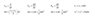# Overlooking something, Need Help

• timpn86
In summary, the first conversation is about an Olympic diver stepping off a 10m high diving board and coming to rest in the water 1.7s after entering it. The task is to determine the velocity at which the diver hits the water and the average acceleration of the diver in the water. The second conversation is about a baseball being dropped and observed to pass a 3.0ft long open window in 0.20s. The task is to find the velocity at the top and bottom of the window, and the height from which the object was dropped.

## Homework Statement

2. An Olympic diver steps off of a 10. m high diving board, plunges into the water and comes to rest beneath the surface of the water 1.7 s after entering it. a) Determine the velocity at which the diver hits the water. b) Determine the average acceleration of the diver in the water.

## Homework Equations

i got to determine the final velocity at which the swimmer reaches the water that in turn will be the initial velocity at which the swimmer enters the water.

equations are attached

Falling
vi = 0 m/s
vf =
a = 9.8 m/s2
t =
s = 10m

In water

Vi =
Vf = 0 m/s
a =
t = 1.7 s
s =

## Homework Statement

A baseball is observed to drop downwards past a 3.0 ft long open window in 0.20 s. a) Find its velocity at the top of the window. b) Find its velocity at the bottom of the window. c) Find how high above the top of the window the object was dropped.

## Homework Equations

equations attached

## The Attempt at a Solution

3ft x .3048 = .9144m

From Drop
vi = 0m/s
vf =
a = 9.8m/s2
t =
s =

Top of Window
vi =
vf =
a = 9.8m/s2
t = .2s
s = .9144m

i was able to finish all 7 other problems on the page but these 2. What am i overlooking?

#### Attachments

•equations.jpg
8.3 KB · Views: 314
Last edited:
Hi timpm,welcome to PF.
Since you have solved the rest of the problem, show some attempt of these two problems. Let me see wher you are stuck.

for first problem, i think you are proceeding in right direction. since height 10m is given, you can find the final velocity before striking water. let it be V.

so avg. acceleration is V/1.7

haha thanks rl.bhat... upon reviewing the problem thurther i was able to find what i missed.